# Subtract two 3-digit numbers

## Learning focus

In this lesson, you will learn how to subtract two three-digit numbers.

The lesson includes:

• one catch-up quiz
• one learning summary
• one video
• two interactive activities
• two worksheets

# Quiz

Test your knowledge of subtracting two 3-digit numbers in this quick quiz.

# Learn

Do you know how to subtract numbers from each other? Find out by watching this short animation featuring a terror from the deep from KS2 Maths.

## Checklist

When subtracting 3 digit numbers it is useful to use this checklist:

• Write the numbers in columns. Separate the numbers into ones, tens and hundreds. Always start with the ones first.

• Estimate first and check afterwards. Estimate a rough answer first, then work out your actual answer.

• Subtraction is the opposite to addition. So subtraction can always be checked by adding. Check that 182 - 37 = 145 by doing the sum: 145 + 37 = 182

• Look out for these words in problems: take away, difference, less than, minus, decrease, fewer than, reduce. They all mean subtraction.

## Column subtraction

The column method is a great way to do subtraction.

## Example 1

• What is 548 - 125?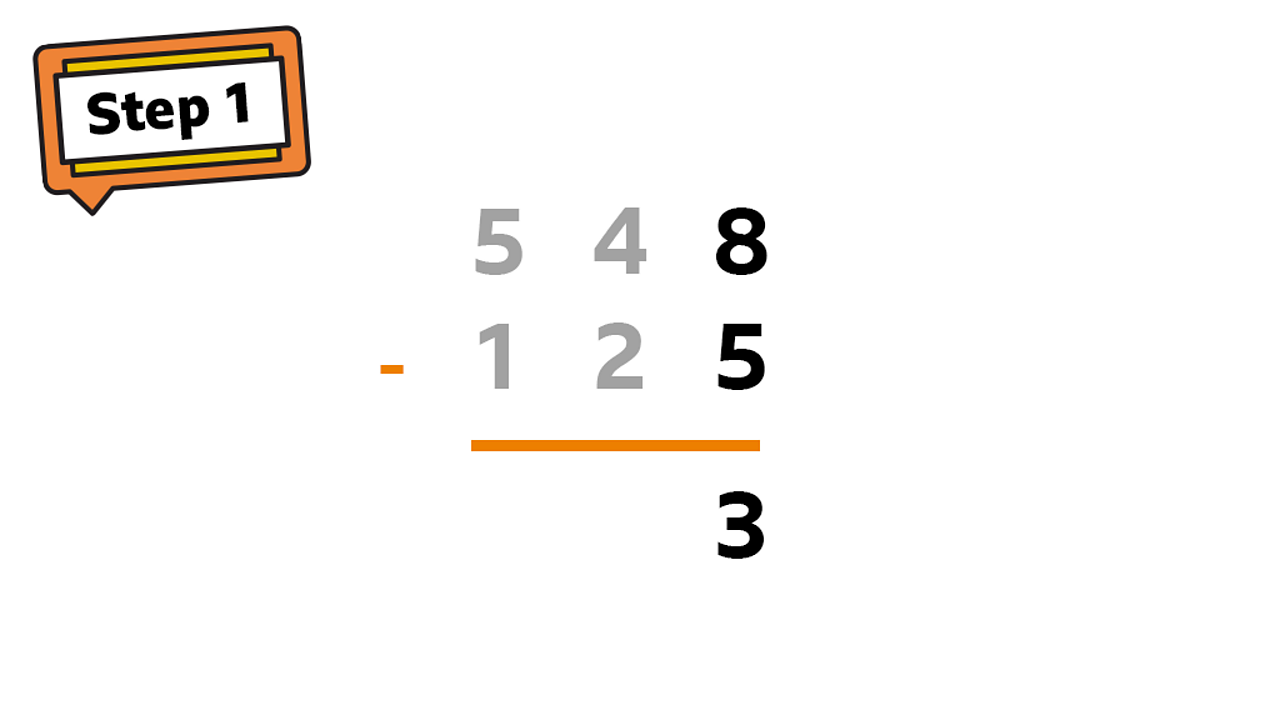Start from the ones column: 8 - 5 = 31 of 4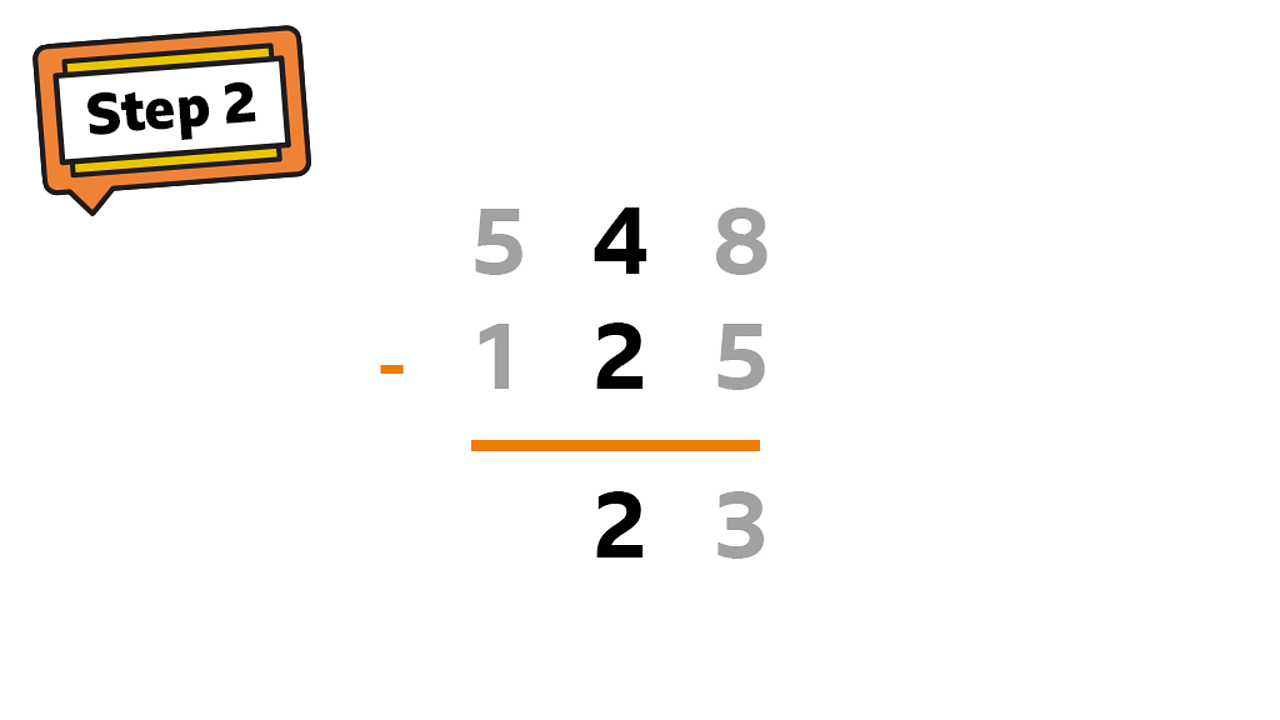In the tens column: 40 - 20 = 20. 2 Is written in tens column as this is worth 202 of 4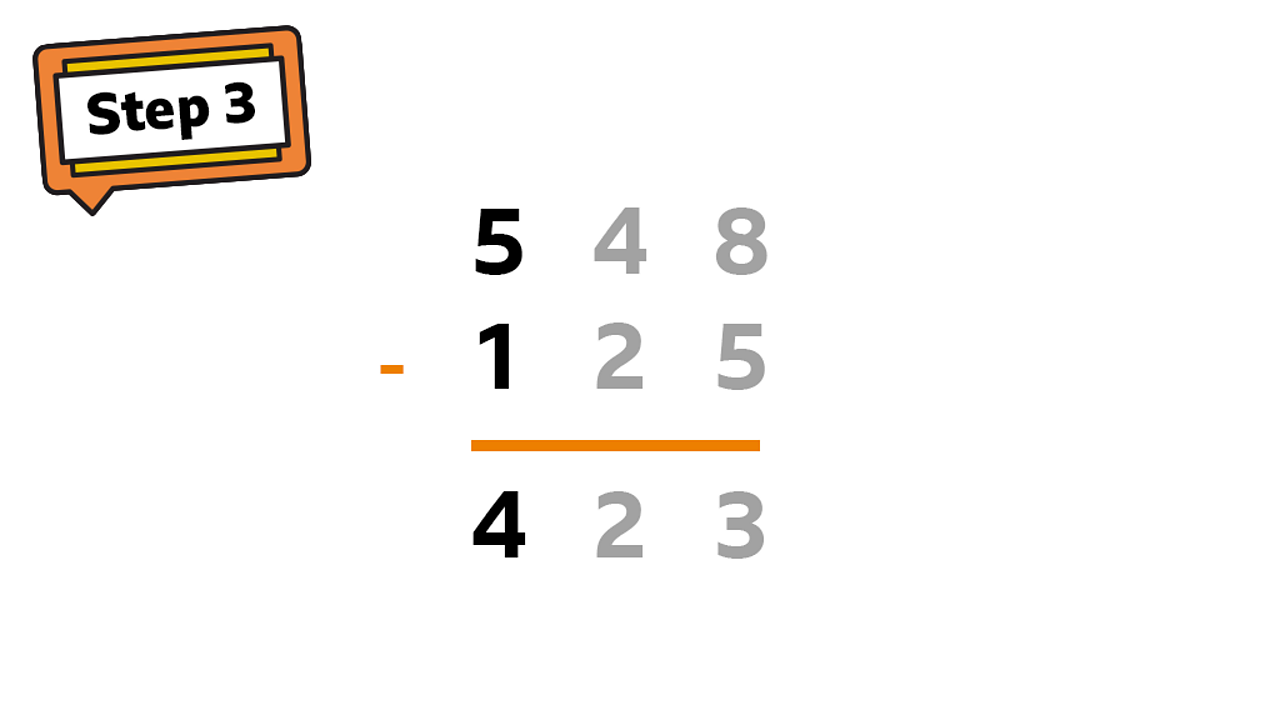In the hundreds column: 500 - 100 = 400. 4 is written in the hundreds column as this is worth 4003 of 4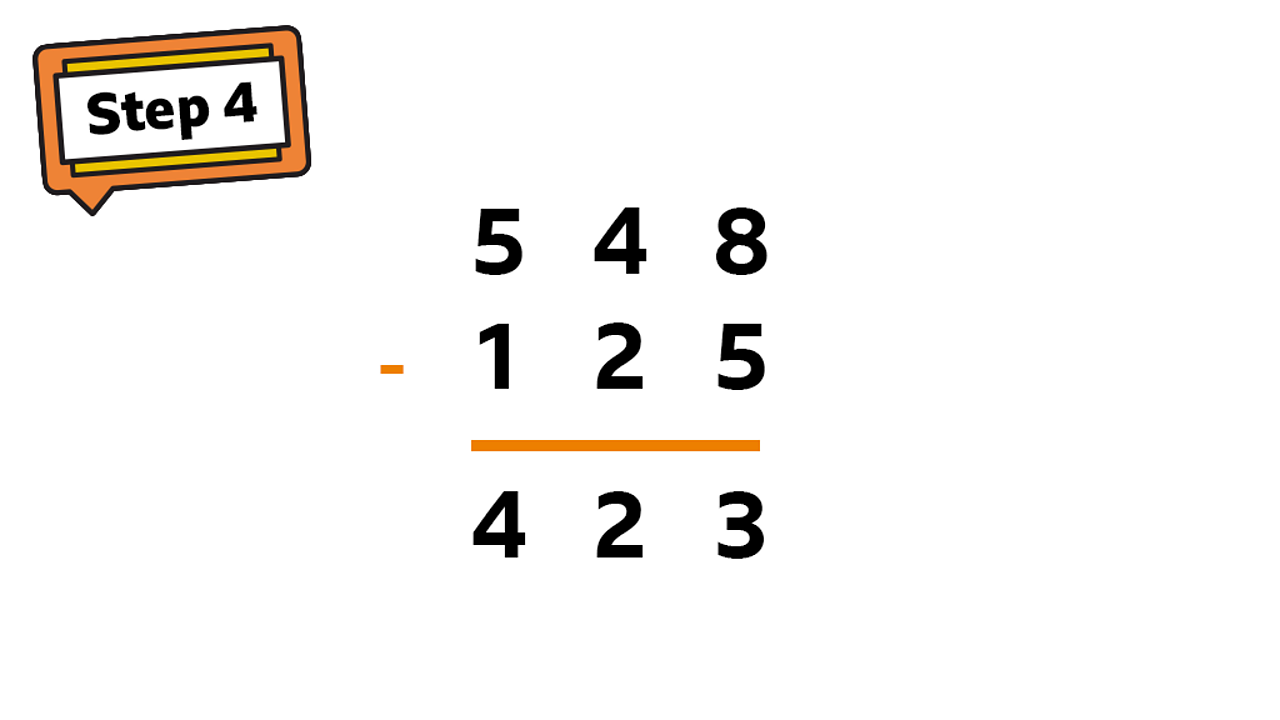The finished subtraction - 4234 of 4

## Example 2

• What is 343 - 237?

When using a place value column to subtract two large numbers, sometimes the second number will be larger than the first. This means it cannot be directly subtracted. You need to exchange a digit from the next column.

This example shows how it works: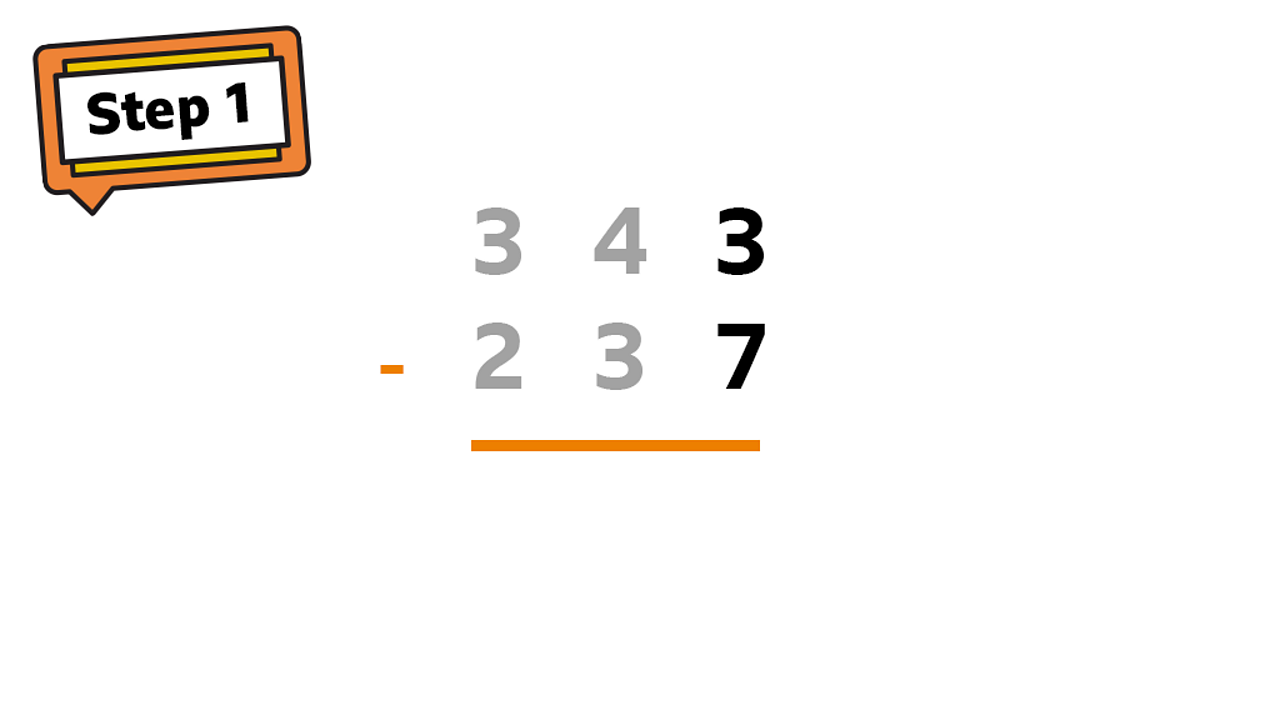Start from the ones column: 3 - 71 of 5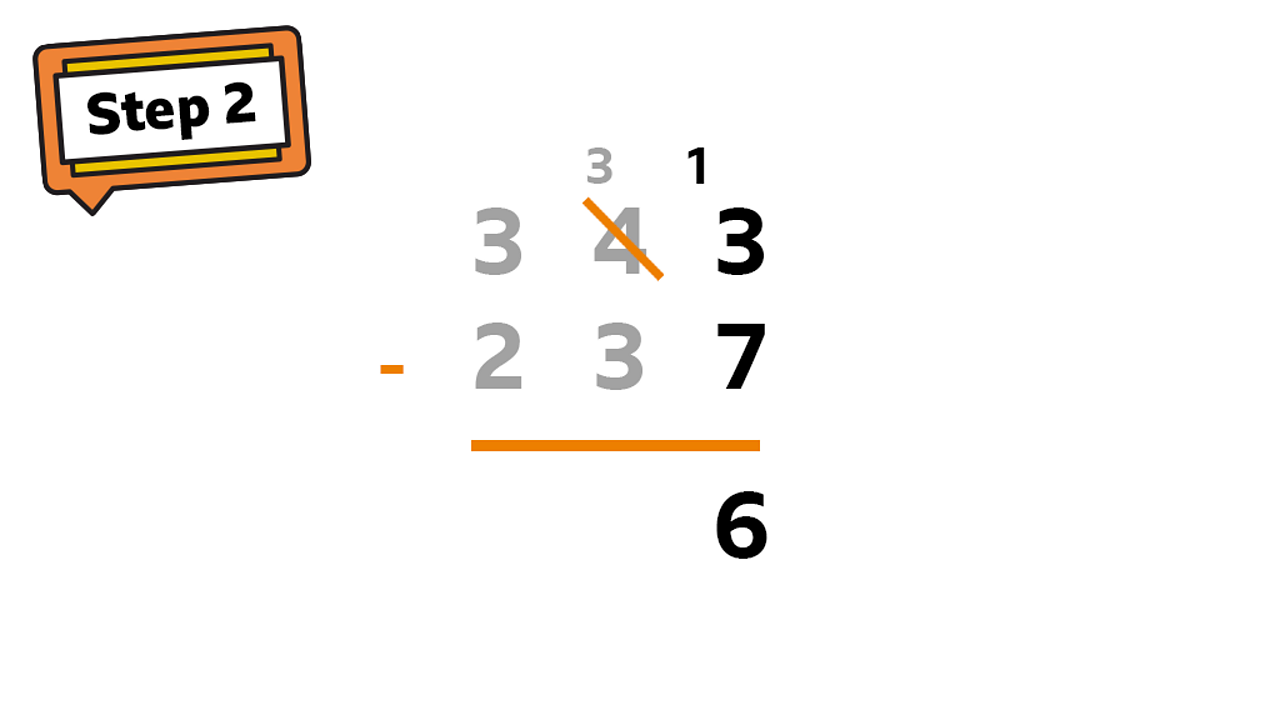It is not possible to subtract 7 from 3 so exchange one ten from the tens column for ten units: 13 - 7 = 62 of 5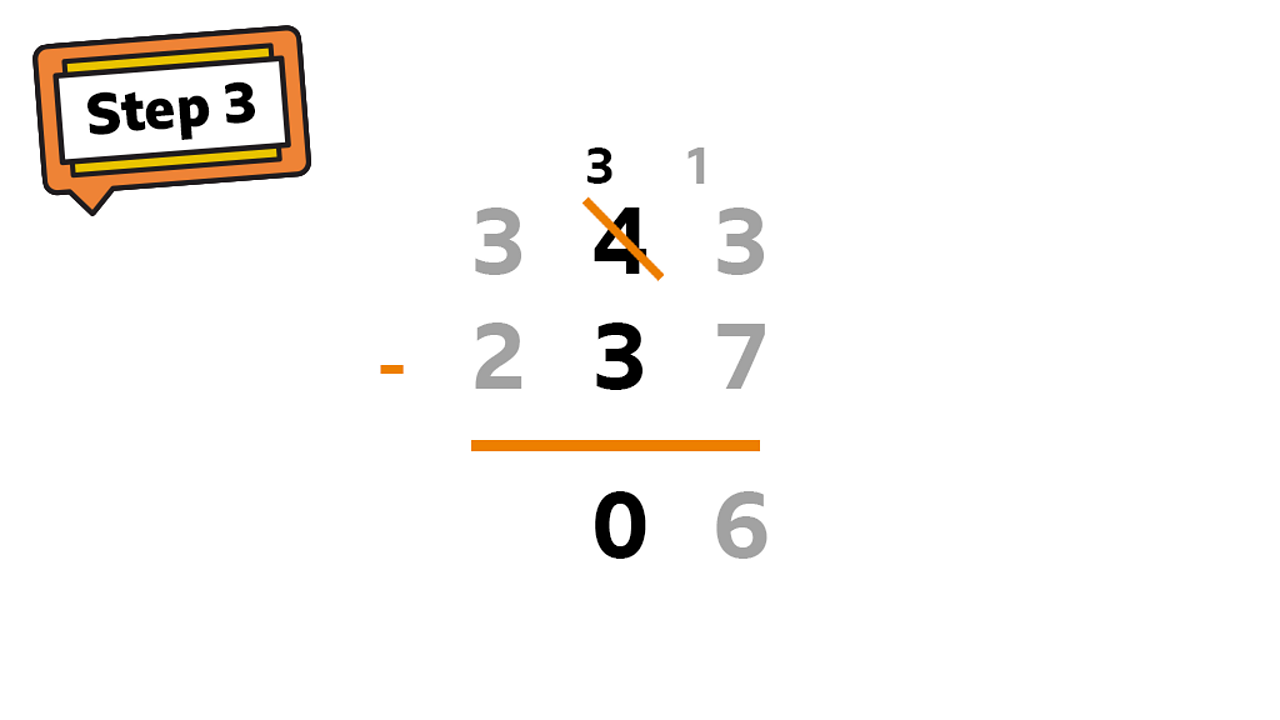In the tens column, one 10 has been exchanged so 40 becomes 30: 30 – 30 = 03 of 5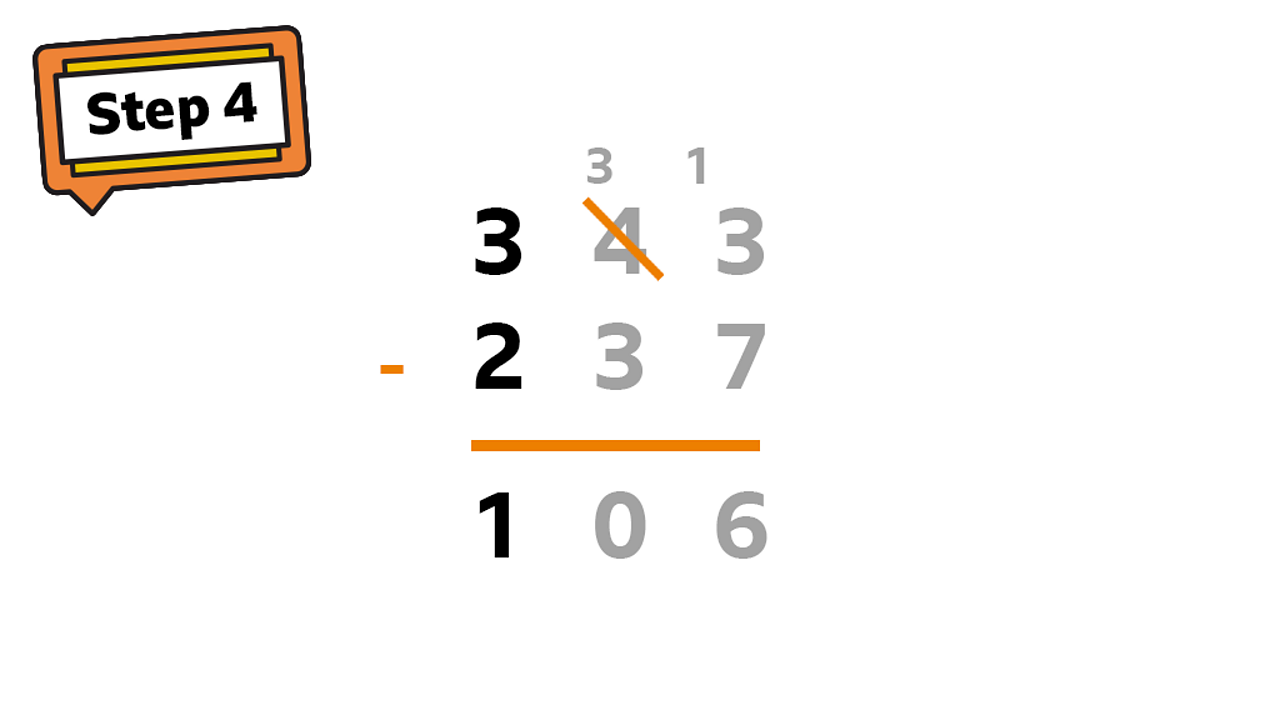In the hundreds column: 300 - 200 = 100. 1 is written in the hundreds column as this is worth 1004 of 5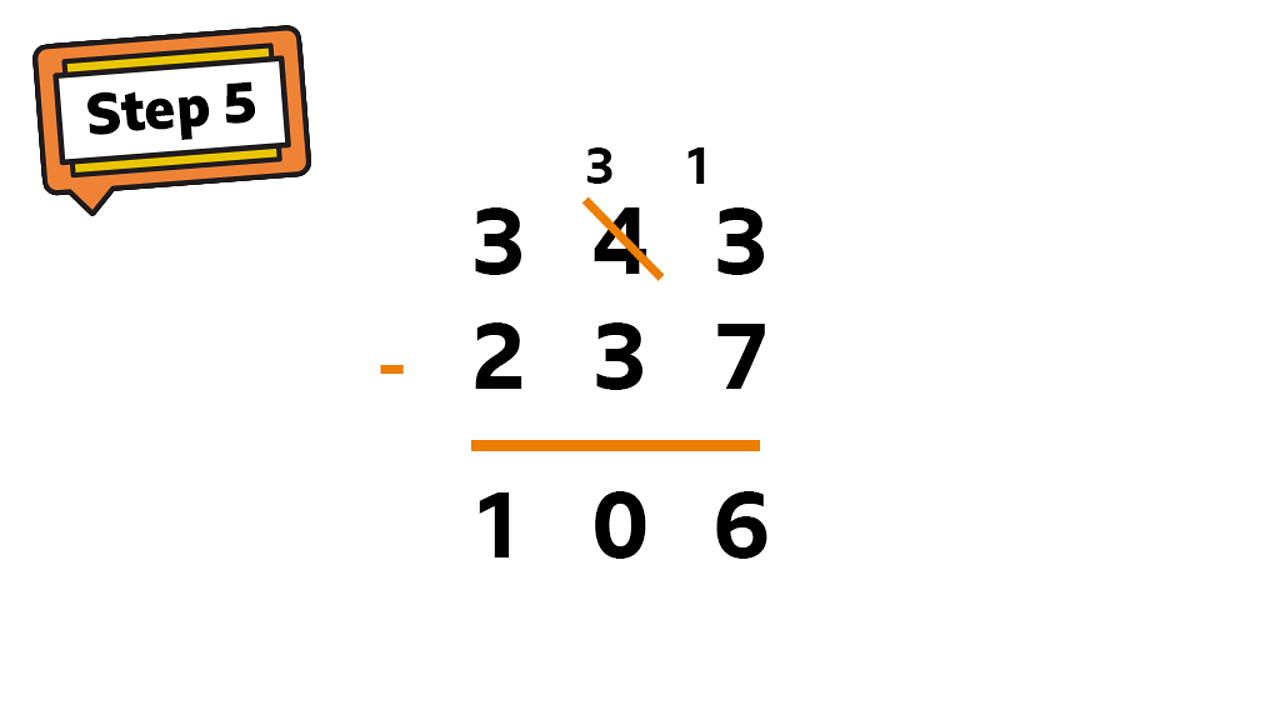The finished subtraction - 1065 of 5

The column method can be applied to help you solve word problems. Remember to look for the words that mean subtraction.

## Example 3

• Lottie has £628 to spend. She buys a new bike which costs £446. How much money does Lottie have left?

The word left tells you that this is a subtraction problem.

# Practise

## Activity 1

Simple subtraction

Click on the image below to see if you can subtract the numbers correctly.

## Activity 2

Some more simple subtraction

Click on the image below to see if you can subtract the numbers correctly.